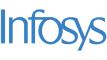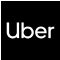New update is available. Click here to update.
Last Updated: 4 Jan, 2021

# Longest Common Subsequence

Moderate+40 more companies

## Problem statement#### A String ‘a’ is a subsequence of a String ‘b’ if ‘a’ can be obtained from ‘b’ by deletion of several (possibly, zero or all) characters. A common subsequence of two Strings is a subsequence that is common to both Strings.

##### Input Format :
``````The first line contains an integer ‘T’ denoting the number of test cases. Then each test case follows.

The first input line of each test case contains two space-separated Strings “STR1” and “STR2” representing two Strings.
``````
##### Output Format :
``````For each test case, print the length of the longest common subsequence.

Print the output of each test case in a separate line.
``````
##### Note:
``````You are not required to print the expected output; it has already been taken care of. Just implement the function.
``````
##### Constraints :
``````1 <= T <= 50
1 <= |STR1| <= 10^2
1 <= |STR2| <= 10^2

Where |STR1| and |STR2| denote the length of “STR1” and “STR2” respectively.

Time Limit: 1 sec
``````## Approaches

### 01 ApproachThe basic idea of this approach is to break the original problem into sub-problems. Let us assume we want to find the length of the longest common subsequence of “STR1” and “STR2” whose length is ‘N’ and ‘M’ respectively.

Now, let us define a recursive function

LCS(Int I, int J, string STR1, string STR2)

Which returns the length of the longest common subsequence of string STR1[0: I-1] and STR1[0: J-1] where STR1[l: R] denotes the substring of “STR1” having characters from index ‘l’ to index ‘R’.

Now, consider the following steps :

1. If ( I == 0 ) i.e. “STR1” is empty then, the length of the LCS will be 0. So return 0.
2. Similarly, if ( J == 0 ) return 0.
3. Now if ( STR1[I-1] == STR2[J-1] ) which means the last character of both string matches then it is optimal to include the current character into the LCS. Therefore, find the LCS of the remaining characters of both strings. So return LCS(I-1, J-1, STR1, STR2) + 1
4. Otherwise, we can not have both STR1[I-1] and STR2[J-1] at the end of the LCS which means either of them can be ignored. Now there are two possibilities, either we ignore STR1[I-1] and get the LCS of the rest of the characters and vice versa. We should choose the option which maximizes the LCS i.e. return max( LCS(I-1, J, STR1, STR2), LCS(I, J-1, STR1, STR2).